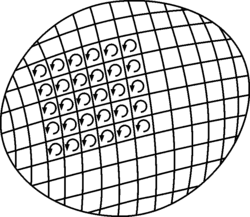Curl is a measure of the tendency of a vector field

$\nabla$$\times$grad(f) is always the zero vector. Can anyone in terms of physical concepts make it intuitive for me, why that is so. I get that the curl is a measure of the tendency of a vector field to rotate or something like that, but couldn't really assemble an understanding just from that.

lanedance
Homework Helper

do you know stokes theorem, which for any vector field g is something like
$$\int_A \nabla \times \textbf{g} \cdot \textbf{dA} = \oint_{\partial A} \textbf{g} \cdot \textbf{dr}$$

it converts an integral over a surface into an inetgral over the boundary. no conside $g = \nabla f$, any closed path integral will be zero - why?

lanedance
Homework Helper

also worth understanding conservative vector fields here

Physically, I'm not sure, but isn't mathematically enough ?
grad(f) is $\nabla$f so you take the vector product of a vector ($\nabla$) with one // to itself ($\nabla$f) so it will be 0 by definition of the vector product. (sorry if it does not help)

cheers..

i'm not especially sure on this, but looking at a drawing, i see that a vector field has rotation near a point x if that point is surrounded by closed loops. but then that means there isn't any directed gradient. conversely, if there is a direction for the gradient field, then there aren't any closed loops to measure rotation.

I know Stokes theorem, but that's just a mathematical theorem, which I by the end of the day then would want to understand intuitively. I do understand that a conservative field is a vector field, which is the gradient of a scalar field. It is then easily shown that the path integral between 2 points is independent of the road taken.
I just wanted the physical interpretation, and I haven't got it from those answers I'm afraid.
In other words: Further help needed! :)

The gradient 'sort of tells you how f is changing in a certain direction'
the curl, 'sort of tells you how much it changes in an orthogonal direction'
once you have limited the changes in the one direction of the grad, the variation that would go in the orthogonal direction of what is left (the curl of the grad) is, well, zero :)

lanedance
Homework Helper

well stokes theorem tells you the curl is similar to the path integral around a loop as you shrink the loop to zero length (orentated in a certain direction)

the gradient of a function is conservative, so the integral around any closed loop will be zero, and isf c is any point onteh closed loop, you can represent as follows
$$\int_A \nabla \times \nabla f \cdot \textbf{dA} = \oint_{\partial A} \nabla f \cdot \textbf{dr} = f(c)-f(c)=0$$

kai_sikorski
Gold Member

To me this picture helps a lot to understand Stokes theoremIt just shows how if you wanna find the circulation (RHS of stokes theorem) for the larger region, that's the same as adding up the contributions from the smaller rectangles because all the contributions from sides not on the boundary of the region cancel.

So to understand Stokes theorem you just need to understand why it would work for an infinitesimal rectangle. There I'm afraid you just need to write out the terms using a Taylor expansion and see that it works. Some things don't necessarily have a nice intuitive explanation.